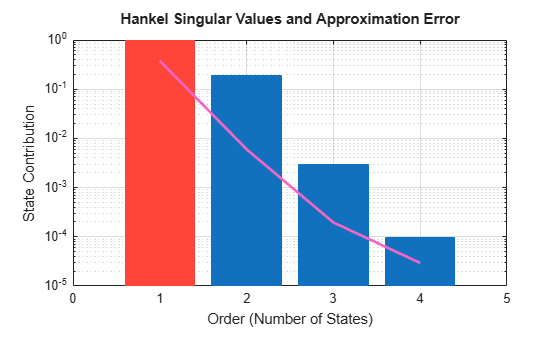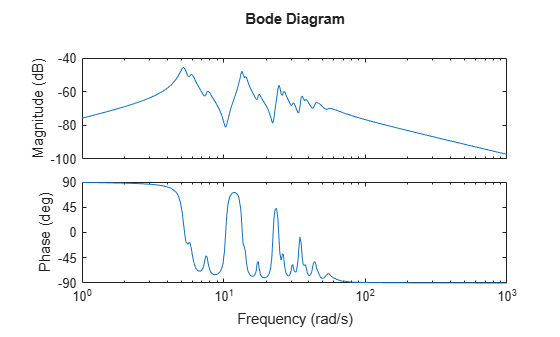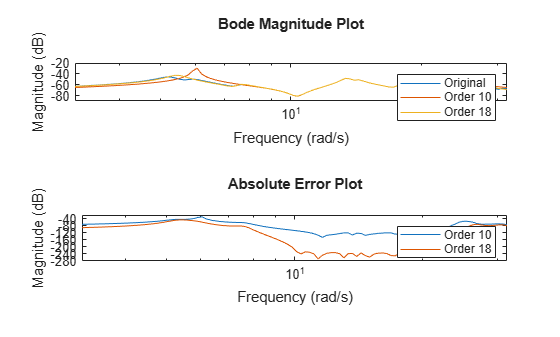# balred

Model order reduction

## Syntax

```rsys = balred(sys,ORDERS) rsys = balred(sys,ORDERS,BALDATA) rsys = balred(___,opts) ```

## Description

`rsys = balred(sys,ORDERS)` computes a reduced-order approximation `rsys` of the LTI model `sys`. The desired order (number of states) for `rsys` is specified by `ORDERS`. You can try multiple orders at once by setting `ORDERS` to a vector of integers, in which case `rsys` is a vector of reduced-order models. `balred` uses implicit balancing techniques to compute the reduced- order approximation `rsys`. Use `hsvd` (Control System Toolbox) to plot the Hankel singular values and pick an adequate approximation order. States with relatively small Hankel singular values can be safely discarded.

When `sys` has unstable poles, it is first decomposed into its stable and unstable parts using `stabsep` (Control System Toolbox), and only the stable part is approximated. Use `balredOptions` (Control System Toolbox) to specify additional options for the stable/unstable decomposition.

This command requires Control System Toolbox™ license. `sys` can only be an identified state-space model (`idss`). The reduced-order model is also an `idss` model.

`rsys = balred(sys,ORDERS,BALDATA)` uses balancing data returned by `hsvd` (Control System Toolbox). Because `hsvd` does most of the work needed to compute `rsys`, this syntax is more efficient when using `hsvd` and `balred` jointly.

`rsys = balred(___,opts)` computes the model reduction using options that you specify using `balredOptions` (Control System Toolbox). Options include offset and tolerance options for computing the stable-unstable decompositions. There also options for emphasizing particular time or frequency intervals. See `balredOptions` (Control System Toolbox) for details.

Note

The order of the approximate model is always at least the number of unstable poles and at most the minimal order of the original model (number `NNZ` of nonzero Hankel singular values using an eps-level relative threshold)

## Examples

collapse all

Compute a reduced-order approximation of the system given by:

`$G\left(s\right)=\frac{\left(s+0.5\right)\left(s+1.1\right)\left(s+2.9\right)}{\left(s+{10}^{-6}\right)\left(s+1\right)\left(s+2\right)\left(s+3\right)}.$`

Create the model.

`sys = zpk([-.5 -1.1 -2.9],[-1e-6 -2 -1 -3],1);`

Exclude the pole at $s=1{0}^{-6}$ from the stable term of the stable/unstable decomposition. To do so, set the `Offset` option of `balredOptions` to a value larger than the pole you want to exclude.

`opt = balredOptions('Offset',.001,'StateElimMethod','Truncate');`

Compute a second-order approximation.

`rsys = balred(sys,2,opt);`

Compare the responses of the original and reduced-order models.

`bodeplot(sys,rsys,'r--')`Reduce a high-order model with a focus on the dynamics in a particular frequency range.

Load a model and examine its frequency response.

```load('highOrderModel.mat','G') bodeplot(G)````G` is a 48th-order model with several large peak regions around 5.2 rad/s, 13.5 rad/s, and 24.5 rad/s, and smaller peaks scattered across many frequencies. Suppose that for your application you are only interested in the dynamics near the second large peak, between 10 rad/s and 22 rad/s. Focus the model reduction on the region of interest to obtain a good match with a low-order approximation. Use `balredOptions` (Control System Toolbox) to specify the frequency interval for `balred`.

```bopt = balredOptions('StateElimMethod','Truncate','FreqIntervals',[10,22]); GLim10 = balred(G,10,bopt); GLim18 = balred(G,18,bopt);```

Examine the frequency responses of the reduced-order models. Also, examine the difference between those responses and the original response (the absolute error).

```subplot(2,1,1); bodemag(G,GLim10,GLim18,logspace(0.5,1.5,100)); title('Bode Magnitude Plot') legend('Original','Order 10','Order 18'); subplot(2,1,2); bodemag(G-GLim10,G-GLim18,logspace(0.5,1.5,100)); title('Absolute Error Plot') legend('Order 10','Order 18');```With the frequency-limited energy computation, even the 10th-order approximation is quite good in the region of interest.

## Alternative Functionality

### App

Model Reducer (Control System Toolbox)

Reduce Model Order (Control System Toolbox)

## Compatibility Considerations

expand all

Behavior changed in R2017b

## References

 Varga, A., "Balancing-Free Square-Root Algorithm for Computing Singular Perturbation Approximations," Proc. of 30th IEEE CDC, Brighton, UK (1991), pp. 1062-1065.

### Topics

Introduced before R2006a

## Support평가판 신청